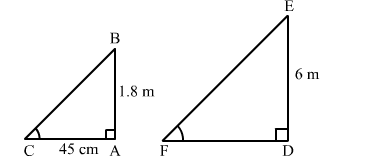# A vertical stick 1.8 m long casts a shadow 45 cm long on the ground. At the same time, what is the length of the shadow of a pole 6 m high?

Question:

A vertical stick 1.8 m long casts a shadow 45 cm long on the ground. At the same time, what is the length of the shadow of a pole 6 m high?
(a) 2.4 m
(b) 1.35 m
(c) 1.5 m
(d) 13.5 m

Solution:

(c) 1.5 mLet AB and AC be the vertical stick and its shadow, respectively.
According to the question:
AB = 1.8 m
AC = 45 cm = 0.45 m
Again, let DE and DF be the pole and its shadow, respectively.
According to the question:
DE = 6 m
DF = ?
Now, in right-angled triangles ABC and DEF, we have:

$\angle B A C=\angle E D F=90^{\circ}$

$\angle A C B=\angle D F E \quad$ (Angular elevation of the Sun at the same time)

Therefore, by AA similarity theorem, we get:

$\triangle A B C \sim \triangle D E F$

$\Rightarrow \frac{A B}{A C}=\frac{D E}{D F}$

$\Rightarrow \frac{1.8}{0.45}=\frac{6}{D F}$

$\Rightarrow D F=\frac{6 \times 0.45}{1.8}=1.5 \mathrm{~m}$